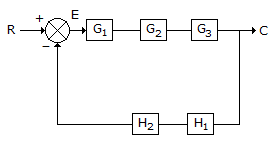Courses

# Test: Instrumentation & Process Control - 4

## 25 Questions MCQ Test Instrumentation and Process Control | Test: Instrumentation & Process Control - 4

Description
This mock test of Test: Instrumentation & Process Control - 4 for Electrical Engineering (EE) helps you for every Electrical Engineering (EE) entrance exam. This contains 25 Multiple Choice Questions for Electrical Engineering (EE) Test: Instrumentation & Process Control - 4 (mcq) to study with solutions a complete question bank. The solved questions answers in this Test: Instrumentation & Process Control - 4 quiz give you a good mix of easy questions and tough questions. Electrical Engineering (EE) students definitely take this Test: Instrumentation & Process Control - 4 exercise for a better result in the exam. You can find other Test: Instrumentation & Process Control - 4 extra questions, long questions & short questions for Electrical Engineering (EE) on EduRev as well by searching above.
QUESTION: 1

Solution:
QUESTION: 2

Solution:
QUESTION: 3

### What is the overall transfer function (C/R) of the following block diagram if G = G1. G2. G3 and H = H1.H2.Solution:
QUESTION: 4

Pick out the most suitable instrument for measuring temperature in the range of-40 to 425�C.

Solution:
QUESTION: 5

Accurate temperature measurement performance of a radiation pyrometer can not be affected, if the

Solution:
QUESTION: 6

Relationship between absorption/evolution of heat at the thermocouple junctions and the current flow in the circuit is given by __________ effect.

Solution:
QUESTION: 7

Configuration of Bourdon spring tube is never made of __________ shape.

Solution:
QUESTION: 8

Thermodynamic celcius scale of temperature measurement is

Solution:
QUESTION: 9

In a closed loop system, the process to be controlled is an integrating process with transfer function l/2s. The controller proposed to be used in an integral controller with transfer function 1/T1s. When a step change in set point is applied to such a closed loop system, the controlled variable will exhibit

Solution:
QUESTION: 10

According to Bode stability criterian, a system is unstable, if the open loop frequency response exhibits an amplitude ratio exceeding unity at frequency for which phase lag is

Solution:
QUESTION: 11

Resistance of a gas in a vessel is given by (where, P = pressure, V = volume of the vessel, n = no. of moles of the gas, R = gas constant)

Solution:
QUESTION: 12

Measurement of pressure in ammonia reactor is done by

Solution:
QUESTION: 13

The fluid used in hydraulic controller is

Solution:
QUESTION: 14

Which of the following thermocouple materials does not contain nickel ?

Solution:
QUESTION: 15

Flow rate of a river is measured by a/an

Solution:
QUESTION: 16

Flow rate of those fluids which are insensitive to changes in their density, viscosity or flow velocity profile can be best measured by a

Solution:
QUESTION: 17

Identify an unbounded input from inputs whose transfer functions are given below

Solution:
QUESTION: 18

"A control system is unstable, if the open loop frequency response exhibits an amplitude ratio exceeding unity at the crossover frequency." This is __________ criterion.

Solution:
QUESTION: 19

Feed forward controller accounts for the __________ changes.

Solution:
QUESTION: 20

Which thermocouple can be used to measure a temperature of around 1400�C ?

Solution:
QUESTION: 21

Which of the following filled system expansion thermometer has the capability to measure the lowest temperature ?

Solution:
QUESTION: 22

Concentration of sugar solution can be determined by the

Solution:
QUESTION: 23

In a single tank system, the transfer function of __________ to inlet flow rate is 1/TS+1.

Solution:
QUESTION: 24

Which of the following can not measure a temperature of 1600�C ?

Solution:
QUESTION: 25

Which of the following instruments is not used for measuring sub-zero (<0°) temperatures ?

Solution: